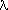# Longitude Systems and Conversion

P1 = reference rotational period of system #1
P2 = reference rotational period of system #2
L1(t-t0) is a known system #1 longitude relative to time t0
L2(t-t0) is the desired longitude value in system #2 relative to time t0
L2(t-t0) = L1(t-t0) + L21 + (t-t0) · [ (24 · 360/P2) - (24 · 360/P1) ]
L21 is a reference longitude. It is defined as:
L21= L2(t=t0) - L1(t=t0)

Jupiter

SI = 9h 50m 30.003s = 877.90°/24h. Used for equatorial motions.
SII = 9h 55m 40.632s = 870.27°/24h. Used for mid-latitudes and GRS.
SIII = 9h 55m 29.711s ± 0.04s = 9.9249197h ± 0.000011h = 870.536°/24h ± 0.000966°/24hI = 67.10 + 877.900 · (t-t0)II = 43.30 + 870.270 · (t-t0)III = 284.95 + 870.536 · (t-t0)(SIII) =(SI) + 217.85 - 7.364 · (t-t0)(SIII) =(SII) + 241.65 + 0.266 · (t-t0)
where t0 = 2451545.0 TDB or Jan. 1.5, 2000.
UIII(SI) = 106.35 m/s
UIII(SII) = -3.4 m/s

Saturn

SIII = 10h 39m 22.4s ± 7s = 10.656222h ± 0.00194h = 810.7939024°/24h ± 0.148°/24hIII = 38.90 + 810.793902 · (t-t0)I = 227.2037 + 844.3 · (t-t0)(SIII) =(SI) + 171.6963 - 33.5061 · (t-t0)
UIII(SI) = 410.36 m/s

For the Voyager encounters the reference time should be set to the closest approach time. Other possibilities are:

 P1 P2 FDS(t=0) L21 Neptune 17.866 16.11 11200.0 -334.5365